Next: Degenerate shell: Seniority model Up: Limiting cases Previous: Weak-pairing limit,

### Strong-pairing limit,In this case, the expansion parameter is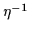. In the zero order, the wave function of an even system with N=2n is the ground state of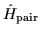; i.e., it corresponds to the state with the maximal quasispin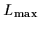=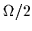and the third component of quasispin L0=(2n-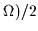. The binding energy is given by the seniority-model expression (see Refs. [27,28] and Sec. 3.2),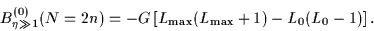(26)

In the quasispin formalism, the single-particle Hamiltonian is a combination of a scalar and a vector operator (with respect to the quasispin group), and this implies the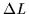=0,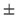1 selection rule for its matrix elements. The expectation value of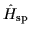in the lowest L=state is: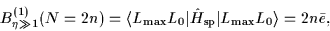(27)

where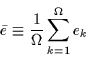(28)

is the average single-particle energy. The second-order correction to the energy is given by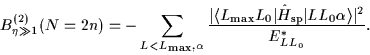(29)

In Eq. (28)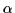denotes all the remaining quantum numbers other than L and L0, and E*LL0 is the unperturbed excitation energy of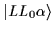. Sincecan only connect the seniority-zero ground state with the seniority-two, L=-1 states at energy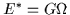, Eq. (29) can be reduced to a simple form;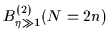=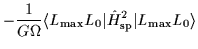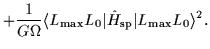(30)

By introducing the variance of single-particle levels,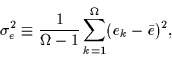(31)

one can derive a simple expression for the second-order correction: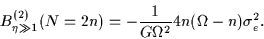(32)

That is, the first- and second-order corrections to the binding energy are given by the first and second moments of the single-particle energy distribution. Note that for the degenerate j-shell, the second-order correction is zero, as expected.

For odd particle numbers, N=2n+1, one should take Lmax=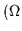-1)/2 and L0=(2n+1-in Eq. (26). Assuming that the odd particle occupies level n+1, this level is removed from the sum of Eqs. (28) and (31), i.e., one needs to consider the remaining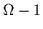levels only: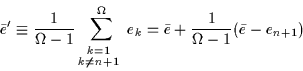(33)

and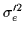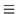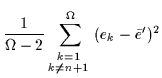=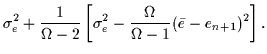(34)

The resulting corrections to the binding energies can be written as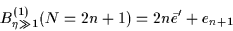(35)

and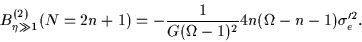(36)

The zero-order expressions for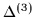and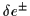in the strong pairing limit are given in Sec. 3.2. By adding the zero- and first-order contributions to the binding energy, one obtains the strong-pairing-limit expressions for: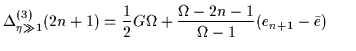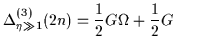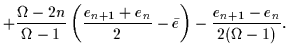(38)

It can easily be shown that the first-order correction to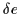vanishes. Consequently, the seniority-model expressions discussed below give a good approximation to the single-particle energy-splitting filters (7) and (8).Next: Degenerate shell: Seniority model Up: Limiting cases Previous: Weak-pairing limit,
Jacek Dobaczewski
2000-03-09